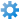# How to calculate roof area using a Custom Column formula | Revu 2017 & below

A number of users have asked if Revu’s built-in measurement tools are able to calculate the area of a roof from a plan view. This calculation is important because it can greatly affect your estimates, the air circulation in a room, and more.

The Custom Columns in Revu’s Markups tab supports formulas and math constants, functions and operators. This makes it possible for you to use more complex formulas that will come in handy when performing roof calculations. These new functions include sin, cos, tan, asin, acos, atan, sqrt, log and ln. The values of Pi and e are also supported.

### Getting started

First, you need to know two specific values in order to calculate the area of a roof: (1) the area of the roof in plan view and (2) the angle of the roof pitch. These two values can be calculated to provide the actual roof area.

Two User Defined Columns need to be created. The first is for the roof angle and the second is a formula that calculates the area.

### Create the User Defined Columns

1. Open the PDF in Revu.
2. Press Alt+L to display the Markups list.
3. Click the Manage Columnsbutton in the Markups list toolbar.
4. Click the Custom Columns tab when the Manage Columns window displays.
6. Type Roof Angle for the Name.
7. Select Number in the Type drop-down list.
8. Click OK.
10. Type Roof Area for the Name.
11. Select Formula in the Type drop-down list.
12. Type or paste the following formula into the Expression field:
`Area / cos([Roof Angle] * pi / 180)`
13. Click OK twice to close the Add Column and Manage Columns windows.

Here’s how the window will look when creating the Roof Area formula:Here is the dialog you should see after both new columns are created:### Measure the Roof

1. Press Alt+M to open the Measurements tab.
2. Click the Anglebutton in the toolbar.
3. Measure the angle of the roof in the elevation view.
4. Click the Areabutton in the toolbar.
5. Measure the area of the roof in the plan view. The Area value that is displayed is incorrect, and will not be the final area.
6. Press Alt+L to open the Markups list.
7. In the Markups list, double-click in the Roof Angle column of the Area measure.
8. Type the roof angle from the Angle measure and press the enter key.
9. The Roof Area column will update and display the calculated roof area.

PDF after the Angle and Area measures are created:Double-click in the Roof Angle column of the Area Measurement, type the roof angle and press enter:The Roof Area is calculated:### Optional

You may want to hide the display of the area value in the plan view to avoid confusion:

1. Select the Area measure.
2. Right-click on the Area measure, then click Properties.
3. Clear the Show Caption check box.

### Explanation of the formula

`Area / cos([Roof Angle] * pi / 180)`
Area is the value of the Area measurement.

Roof Angle is the value in the User Defined Column that you created.

Cos is the math function cosine

Pi is 3.14

Please checkout our video tutorials on PDF Measurement , the Measurement Enhancements in Revu 12 , and Takeoffs and Estimations , in addition to the FAQ section on our Support page, and the Revu Help Guide.

A number of users have asked if Revu’s built-in measurement tools are able to calculate the area of a roof from a plan view. This calculation is important because it can greatly affect your estimates, the air circulation in a room, and more.
The Custom Columns in Revu’s Markups tab supports formulas and math constants, functions and operators. This makes it possible for you to use more complex formulas that will come in handy when performing roof calculations. These new functions include , , , , , , , and . The values of and are also supported.
Getting started
First, you need to know two specific values in order to calculate the area of a roof:
is the value of the Area measurement.
Roof Angle is the value in the User Defined Column that you created.
is the math function cosine
is 3.14

Please checkout our video tutorials on PDF Measurement , the Measurement Enhancements in Revu 12 , and Takeoffs and Estimations , in addition to the FAQ section on our Support page, and the Revu H